Home MonkeyNotes Printable Notes Digital Library Study Guides Study Smart Parents Tips College Planning Test Prep Fun Zone Help / FAQ How to Cite New Title Request

 7.4 Fundamental Theorem of Integral Calculus We have studied the definite integral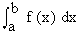as the limit of a sum. Now we will see definite integralas an anti derivative. The evaluation of the definite integral as the "Limit of a sum" is a cumbersome process. (see example 2). Moreover it is not always necessary or practicable to evaluate a definite integral "ab - initio" as the "limit of a sum". For it is possible to prove that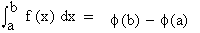provided there exists a function such that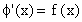. This is given in the fundamental theorem of integral calculus as - Ifregarded as the limit of a sum exists and there exists a function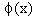such that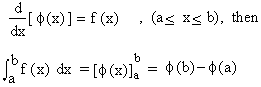The proof of this theorem is beyond the scope of this book. Note that in evaluating, we need not add c to the primitiveof f(x). This is evident from the fact that if we add c. It is automatically eliminated.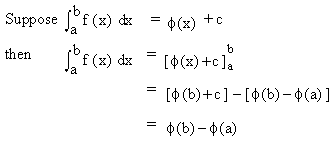This justifies the uniqueness and the adjective "definite" for this type of integral. Now there is a significant relationship between the continuity and differentiation. This fact leads us to say that if a function f (x) is continuous on [a, b] then the definite integral of f (x) on [a, b] exists. Otherwise it may or may not be integrable on [a, b]. In other words, continuity guarantees the existence of the definite integral but its converse is not necessarily true. Index 7.1 Definition 7.2 Definite integral as on an area under curve 7.3 Working rule for evaluation of a definite integral as a limit of a sun 7.4 Fundamental theorem of integrated calculus 7.5 Properties of definite integrals 7.6 Evaluation of definite integral 7.7 Reduction formulas Chapter 8